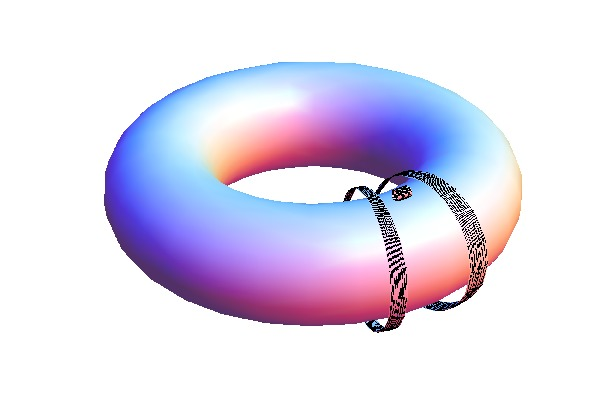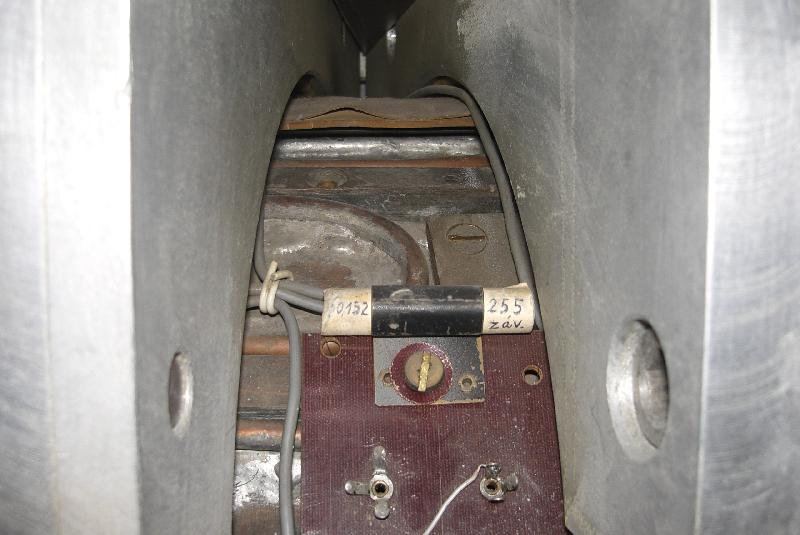--- format: markdown title: Toroidal magnetic field measurements author: Ing. Kateřina Jiráková categories: Diagnostics ... The toroidal magnetic field $B_T$ is measured by a small coil (called the $B_T$ coil) fixed to the tokamak upper outboard side, its axis pointing along the torodial direction. The general principle of magnetic coil measurements is described [here](/Diagnostics/Magnetic/Theory/Magnetic_coil/description). The $B_T$ coil is one of the basic GOLEM diagnostics.
 [](//Diagnostics/Basic/ToroidalMagneticField/Sketch/dascomp.jpg) $B_T$ coil location on the tokamak [](/Diagnostics/Magnetic/SmallCoil4ToroidalMagneticField/cimg7940.jpg) $B_T$ coil
# Coil description The $B_T$ coil is made of 255 turns, each having a diameter of $10 \, \mathrm{mm}$. Its effective area is therefore $0.02 \, \mathrm{m}^2$. Its exact coordinates in the $(R,Z)$ plane are not known but this isn't an issue; see below. The coil voltage is collected by the DAS1 data acquisition system. ## Coil effective area for area-averaged $B_T$ measurements There is a large loop encircling the tokamak chamber in the poloidal direction; its radius is $0.145 \, \mathrm{m}$ and its area is $0.066 \, \mathrm{m}^2$. Even though it is not currently part of the tokamak diagnostic system, it can be used for measurements of $B_T$ averaged over the entire loop area. It was measured that when the $B_T$ coil signal is averaged over this area (considering that $B_T \sim 1/R$), its sensitivity is $5.98 \div 6.06$ times lower than the large loop sensitivity. This implies that the $B_T$ coil effective area is $0.066 \, \mathrm{m}^2 / 6 = 0.011 \, \mathrm{m}^2$ when it's used for area-averaged $B_T$ measurements. # $B_T$ measurement Cannonically the toroidal field $B_T$ is always given at the chamber centre. This is because it falls with the major radius as $B_T \sim 1/R$, and so a fixed measurement location is required to compare between various tokamaks and discharges. Unfortunately the $B_T$ coil is not located at $R=R_0$; rather it is further outside. However, since the chamber doesn't move anywhere and neither does the coil, the ratio $B_{T0}/B_{T,coil}$ is fixed. Many years ago a calibration experiment was conducted where a measuring coil was inserted into the centre of the tokamak chamber. By comparing its signal to the $B_T$ coil signal, the following calibration relation was derived: $$B_{T0}(t) = 70.42 \, \mathrm{T/Vs} \int_0^t V_{coil}(t') \mathrm{d}t'$$ Here $V_{coil}$ is the voltage induced on the $B_T$ coil. The constant $70.42 \, \mathrm{T/Vs}$ [[source]](http://golem.fjfi.cvut.cz/shots/30001/basicdiagn/config.py) is several calibration constants combined into one. It includes both the calibration "induced voltage $V_{coil} \rightarrow$ magnetic field $B_{T,coil}$" (featuring the number of coil turns and the coil effective area) and the calibration "$B_{T,coil} \rightarrow B_{T0}$". # Various links - A basic block diagram of signal processing: [bd_dB_t.pdf](/Diagnostics/Basic/ToroidalMagneticField/BlockDiagram/bd_dB_t.pdf) - Photos of the $B_T$ coil: [/Diagnostics/Basic/ToroidalMagneticField/PhotoGallery/](/Diagnostics/Basic/ToroidalMagneticField/PhotoGallery/) - Alternative, outdated page on $B_T$ measurement: [diagnostics_torcoil](/Diagnostics/Magnetic/SmallCoil4ToroidalMagneticField/diagnostics_torcoil) # To do - find which DAS the $B_T$ coil is plugged into - procure a general magnetic coil signal processing guide